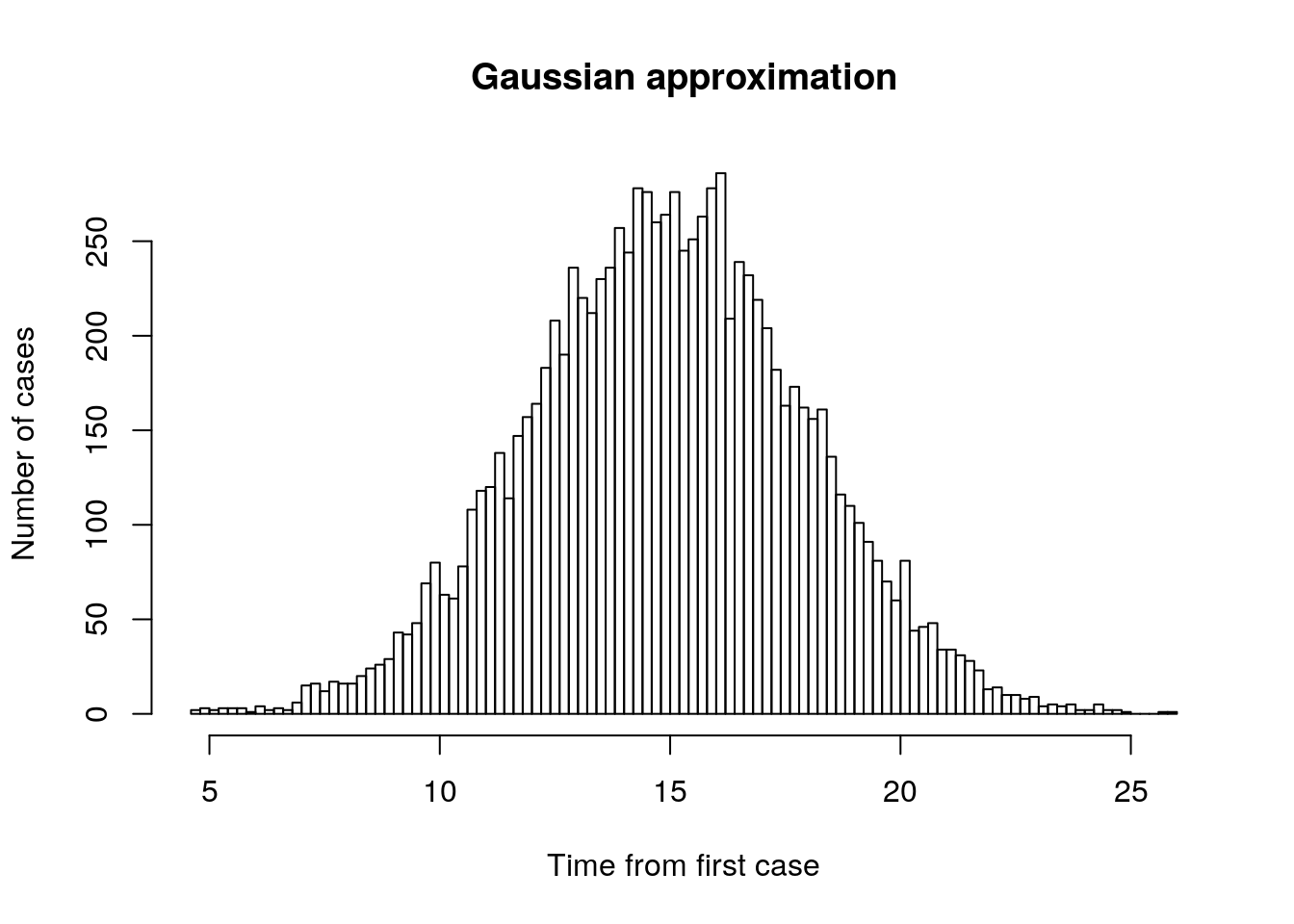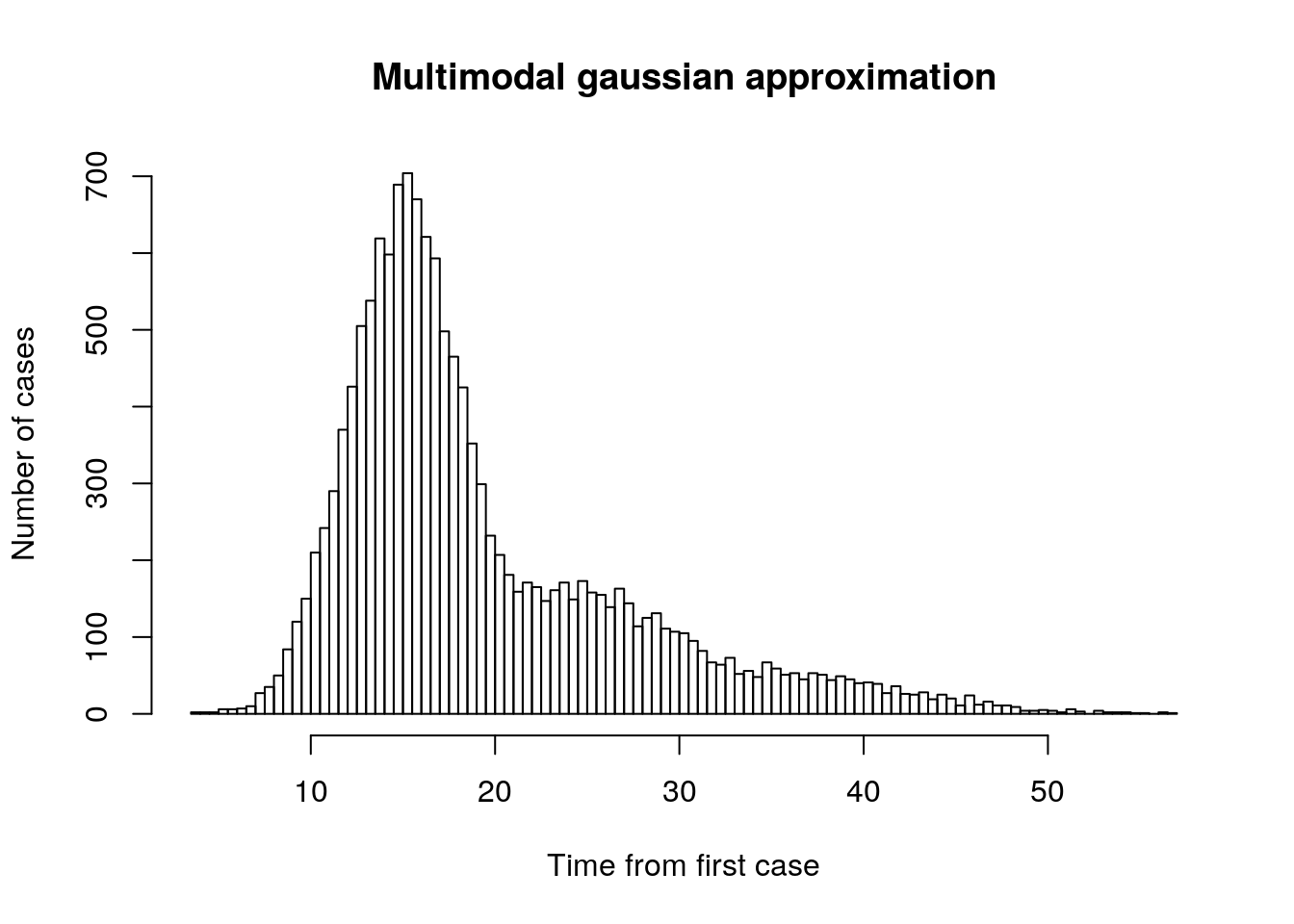When epidemics or pandemics are modelled under a simple SIR framework the issue of asynchronicity in the peaks of individual outbreaks can be overlooked. An epidemic (or pandemic) is in fact a multi-modal mixture model. The infected element of a dynamic SIR model can be very loosely approximated as a Gaussian distribution (It is clearly not Gaussian, but this ĵust serves as a very quick illustration of a general concept) . Let’s depict the shape of a single major outbreak as Gaussian.

t<- rnorm(10000,15,3)
hist(t, breaks =100, xlab="Time from first case", ylab="Number of cases",main="Gaussian approximation")Now, let’s assume that following this outbreak a set of smaller outbreaks occur that are added to the total case numbers. The outbreaks become slightly “flatter” over time due to increased social distancing that lower the peak of the curves.

t1<- rnorm(10000,15,3)
t2<- rnorm(3000,25,4)
t3<- rnorm(1000,35,5)
t4<- rnorm(500,40,6)

t<-c(t1,t2,t3,t4)

hist(t, breaks =100, xlab="Time from first case", ylab="Number of cases",main="Multimodal gaussian approximation")This general pattern is frequently observed in empirical data sets representing areas large enough to have had several outbreaks.

It is easily reproduced. Finding a long tail does not imply that any major alterations to the general SIR modelling framework are required.

To match the empirical data all that is needed is to disaggregate the SIR and then fit it to the multiple outbreaks in each subregion. The epidemic curve becomes the integral over all the separate outbreaks, each one with slightly different times of onset and different parameters, which may be as a result of measures that influence transmission and duration of infection. The shape of the underlying curve for any one of the individual outbreak does not need to change. Each outbreak can still tend to be repressented by an approximately symmetrical curve.